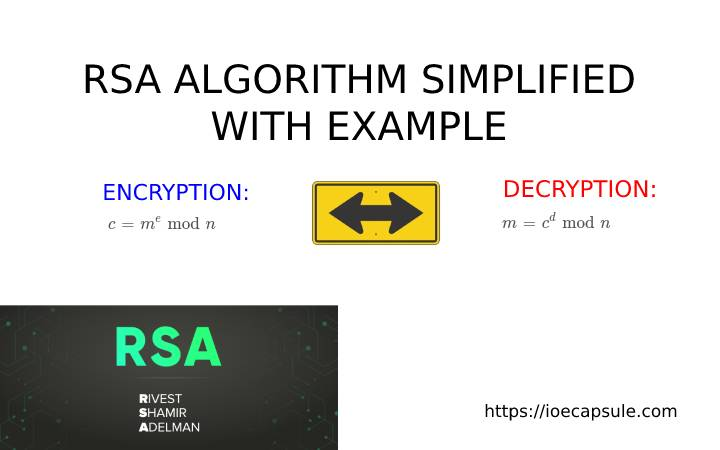### Cryptography – RSA Algorithm Simplified With ExampleAlgorithms

RSA is probably the most commonly used secure cryptographic algorithm. The acronym RSA comes from the surnames of Ron Rivest, Adi Shamir and Leonard Adleman, who publicly described the algorithm in 1977. It’s usage is undeniably the greatest for secure data transmission.

## What is RSA algorithm ?

RSA is an asymmetric cryptographic algorithm, widely used to the purpose of secure data transmission. The asymmetric cryptography means that there will be two different keys, one private and other public, linked via some mathematical function. It is also called a public key algorithm. The public key is meant to be spread over public users and private key is kept at secure location where no one else can access.

## Basic working of RSA

We can take simple browser request for example. Let us consider we are using RSA for performing some request on server. The basic steps that is executed behind the scenes are as follows:

1. The browser sends it’s public key to the server and requests for some service. Let’s say, browser is asking for some data.
2. The server receives the request, generates data, encrypts it with browser’s public key and returns encrypted data as response.
3. The browser receives encrypted data, decrypts it and uses it as required.

Alright, that’s was the basic working. Let’s see the algorithm, more importantly how public key and private key are generated.

## What are the steps of RSA algorithm ?

1. Select two prime numbers. Let’s say we select p = 3 and q = 11.
2. Find the multiplication of p and q as n = p × q .
3. Find the multiplication of p-1 and q-1 as Φ(n) = (p – 1) × (q – 1) .
4. Assume an exponent e such that 1 < e < Φ(n) and e is co-prime of Φ(n). The pair (n, e) is used as public key.
5. Find d such that d×e = 1 mod Φ(n). The pair (n, d) is used as private key.
6. Encryption: If m is the plain text number which is to be encrypted, the encrypted ciphertext c can be calculated as
c = me mod n .
7. Decryption: The encrypted ciphertext c can be decrypted as
m = cd mod n .

These were the actual mathematical operations that happen while generating public/private keys and performing encryption/decryption. To brush up the mind a little, let’s encrypt and decrypt a random word by ourselves.

## Encrypt/Decrypt the word “computer” using RSA algorithm

Alright. We have a word computer to perform encryption and decryption. But before doing so, we need public and private keys. Let’s look back a little to the algorithm part and do similar steps so that we can generate public key and private key number pairs.

The first step is to assume two prime numbers. For simplicity, lets say p = 3 and q = 11. Now, n = p x q gives n = 33 and Φ(n) = (p – 1) x (q – 1) gives Φ(n) = 20. Assume a number for exponent e so that 1 < e < Φ(n) and e is co-prime of Φ(n). Let’s say that number is e = 7. So, we have now a public key pair (n, e) = (33, 7).

That last step left is to find private key pair. For that we need to find d such that d×e = 1 mod Φ(n) . This implies, d = 3. You can calculate however you want, using calculator can be handy. So, we have found our private key pair as (n, d) = (33, 3) .

Now, let’s perform encryption and decryption of word computer . Since, we need numeric value, first we need to assign numerical value to each character in the word. For the sake of simplicity, we can simply put numbers alphabetically. The letter a becomes 1, b becomes 2, c becomes 3, …….., z becomes 26.

For encryption, we will use c = me mod n and for decryption, we will use m = cd mod n . The encrypted and decrypted data can be seen in the following table.

I hope you understood the process. If you are a learner, try other words as well. Implementing on programming language can also boost your understanding. So, if you are a programming guy, try doing so on any language you like.